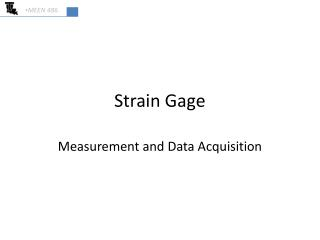DownloadDownload PresentationStrain Gage

# Strain Gage

Télécharger la présentation## Strain Gage

- - - - - - - - - - - - - - - - - - - - - - - - - - - E N D - - - - - - - - - - - - - - - - - - - - - - - - - - -
##### Presentation Transcript

1. MEEN 486 Strain Gage Measurement and Data Acquisition

2. Strain Gage • Electrical component • Bonded to material • Strains as material strains • Resistance changes as gage strains • R=120 W (nominal)

3. Strain Gage • Calibration information • Manufacturer provides “gage factor” or GF • 2.x typical dR/R m=GF dL/L

4. Strain Gage • Equation • Measure R and change in R • Calculate strain (e) • So how do we measure R?

5. Measure R • Fluke DMM • Measure R directly • Agilent DMM • Measure R directly • Data to PC if desired

6. Balance or Deflection • “Measure R” using Wheatstone Bridge • R1 is the strain gage • R2 is a variable resistor • Balanced: • Eo is Galvanometer • Achieve null i • Manual (slow) or feedback • Deflection: • Eo is voltmeter • Direct! Fast!

7. Deflection Bridge • Measure voltage output of bridge • Calculate unknown resistance R1 (strain gage) • Equation • Equation for R1 from measured Eo

8. Deflection Bridge and High-speed DAQ • Connect USB-6008 to Deflection Bridge • Measure voltage • Calculate R1 • 10,000 samples per second • 12 bit • Recall • Resolution • Quantization error – u0

9. LabVIEW Review • Not now • Have NEW tutorial available • MEEN 382 F2010 • Take me about 5 minutes to refresh your memory • BH 118A or install on Laptop (30 day?) • Contact me if you want to use this • or if you think you might…

10. Strain Gage • Let’s try it out • Deflect beam/strain gage • Observe R change • Deflect beam • Observe voltage change on Deflection Bridge • Calculate R1 from Bridge Equation • Calculate e from Strain Gage Equation • How good is the answer? • Hwk to follow…